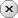### 用户注册### 用户登录### 发表随想# Python机器学习：鸢尾花分类

2023-03-26 作者： Python自学举报

## [python]代码库

```import pandas as pd
import numpy as np
from sklearn.model_selection import train_test_split
from sklearn.neighbors import KNeighborsClassifier

X = iris.data
y = iris.target

X_train, X_test, y_train, y_test = train_test_split(X, y, test_size=0.3, random_state=1)

knn = KNeighborsClassifier(n_neighbors=3)
knn.fit(X_train, y_train)

print("预测结果：", knn.predict(X_test))
print("准确率：", knn.score(X_test, y_test))
```

### 评论须知：

• 1、评论每次加2分，每天上限为30；
• 2、请文明用语，共同创建干净的技术交流环境；
• 3、若被发现提交非法信息，评论将会被删除，并且给予扣分处理，严重者给予封号处理；
• 4、请勿发布广告信息或其他无关评论，否则将会删除评论并扣分，严重者给予封号处理。

### 扫码下载### 输入口令后可复制整站源码